Get inspired by the success stories of our students in IIT JAM MS, ISI  MStat, CMI MSc DS.  Learn More

# Hyperbola & Tangent | ISI MStat 2016 Problem 1 | PSB SampleThis is a beautiful sample problem from ISI MStat 2016 PSB Problem 1. This is based on finding the minimum value of a function subjected to the restriction.

## ISI MStat 2016 Problem 1

Let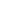be real numbers such that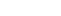. Find the minimum value of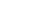and all also find all the points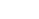where this minimum value is achieved.

## Solution

(a) Using graph

The equation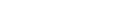represents the equation of rectangular hyperbola with foci are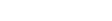and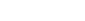.

Now ,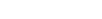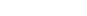, which looks somewhat like this ,

we have to find the the minimum value ofsubject to the restriction that. If we move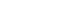alongby varying c , then local minimum can occur at the points where the level curvetouch. Now as both the rectangular hyperbola and |x+y|=c are symmetric about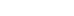for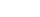and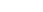, the level curve will touchwhen x+y=c and x+y=-c both are tangent to the curve. And tangents occurs at the foci ofi.e atand.

Hence , the minimum value ofare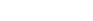and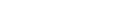both gives the same value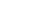.

Therefore , the minimum value ofisand it attains it's minimum atand.

(b) Using Derivative test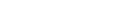as we are given thatLet,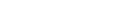then we have to find the minimum value of f(x)

Now ,as the function is not defined at x=0 and also x=0 can't give the minimum value of |x+y| due to the condition that xy=10. So, we will study f(x) for two cases when x>0 and when x<0 .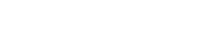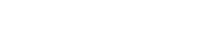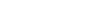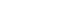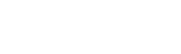So,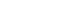forHence f(x) attains it's minimum value atand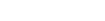and minimum value is.

## Challenge Problem

Let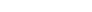be be positive real numbers such that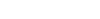. Find the minimum value of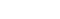.

This is a beautiful sample problem from ISI MStat 2016 PSB Problem 1. This is based on finding the minimum value of a function subjected to the restriction.

## ISI MStat 2016 Problem 1

Letbe real numbers such that. Find the minimum value ofand all also find all the pointswhere this minimum value is achieved.

## Solution

(a) Using graph

The equationrepresents the equation of rectangular hyperbola with foci areand.

Now ,, which looks somewhat like this ,

we have to find the the minimum value ofsubject to the restriction that. If we movealongby varying c , then local minimum can occur at the points where the level curvetouch. Now as both the rectangular hyperbola and |x+y|=c are symmetric aboutforand, the level curve will touchwhen x+y=c and x+y=-c both are tangent to the curve. And tangents occurs at the foci ofi.e atand.

Hence , the minimum value ofareandboth gives the same value.

Therefore , the minimum value ofisand it attains it's minimum atand.

(b) Using Derivative testas we are given thatLet,then we have to find the minimum value of f(x)

Now ,as the function is not defined at x=0 and also x=0 can't give the minimum value of |x+y| due to the condition that xy=10. So, we will study f(x) for two cases when x>0 and when x<0 .So,forHence f(x) attains it's minimum value atandand minimum value is.

## Challenge Problem

Letbe be positive real numbers such that. Find the minimum value of.

This site uses Akismet to reduce spam. Learn how your comment data is processed.

### 2 comments on “Hyperbola & Tangent | ISI MStat 2016 Problem 1 | PSB Sample”

1.Sourav Biswas says:

Yeah, I went through your process and this was absolutely right. Couldn't it be solved without Calculus? I approached in another way. We have the identity (x+y)2 - (x-2)2 = 4xy. Then (x+y)2>= 4xy. that is |x+y|>= 2 root(xy). in our problem, |x+y|>= 2root(10). That is min|x+y| = 2root(10). Equality will occur iff x=y. then, 2|x|= 2root(10) which gives, |x|=root(10). then also |y|=root(10). Remembering the restriction, x=y, we have the required pairs (x,y) as (root10, root10), (-root10, -root10).
I think this process is quite elegant and Time Saving!

1.Srijit Mukherjee says:

Yeah, this one is great and crispy too. 😀 Thanks

### Knowledge Partner